# Trap Error or Replace Error by Specific Value with IFERROR function

We often use Excel formulas in our work life, and we may encounter this situation that the formula throws an error and finally an error like #DIV/0! Is displayed in the cell. In today’s tutorial, we will introduce you the application of Excel IFERROR function to catch errors and replace errors by specific values per your requirement.

In today’s example, if the IFERROR formula returns a normal value, we keep the normal value, and if it throws an error, we will return a specific value or a text to mark the error type.

Using a simple division as an example, the division reports error #DIV/0! if the divisor is zero. We use the following example to show you how to use Excel IFERROR function to return a specific value in Excel worksheet if #DIV/0! error is detected.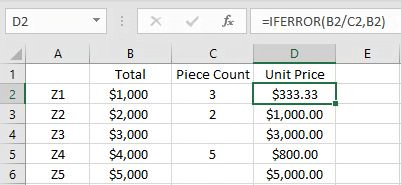In this example, if the cell in Piece Count column is not empty, the formula will return the unit price calculated by Total/Piece; And if the cell in Piece Count column is empty, the formula will return the value saved in Total column instead.

Notes:

In order to get the return value with \$, we need to set the cell format to “Currency” before entering the formula. You can set “Currency” via Home->Number->Number Format dropdown list.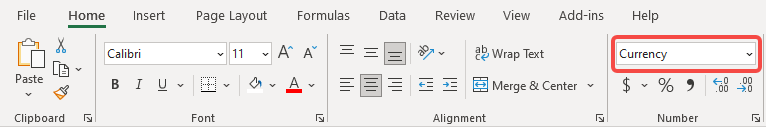## FORMULA

In this example, we have used only the IFERROR function without nesting other functions.

Excel IFERROR function detects if a formula or expression is in error and returns a specific value if the formula evaluates to an error. It returns the result of the formula or expression if there is no error.

Syntax:

`=IFERROR(value, value_if_error) `

In this example, we can directly apply this function with entering our own value and value if error.

`= IFERROR(B2/C2, B2)`

## EXPLANATION

Normally, if we just enter =B2/C2 without applying Excel IFERROR function in unit price column, #DIV/0! error will be displayed if piece count is empty.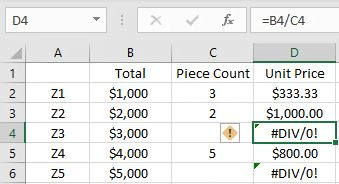After introducing Excel IFERROR function, we can know that if error is thrown by the input expression or formula, we can record the error by setting a specific value (argument “value_if_error”) in IFERROR, then the normal error will be replaced by this value. This value can be a cell reference, a fixed value, a formula, or an error mark like “error code1”.

### RETURN A SPECIFIC VALUE

In this example, we want to get the unit price by Total/Piece Count. We notice that in Piece Count column, some cells are empty, which means that the divisor is empty. In this case, we want to keep their total value as their unit price, so if we encounter an error like this, the total value will be returned by IFERROR.

Calculate B2/C2 in the formula bar:

`=IFERROR(333.333333333333,1000)`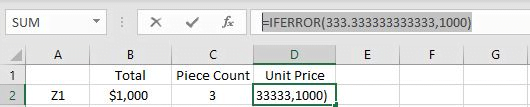Expression B2/C2 returns value 333.3333 and doesn’t throw an error, so the result of the expression is displayed in D2.

Calculate B4/C4 in the formula bar:

`=IFERROR(#DIV/0!,3000)`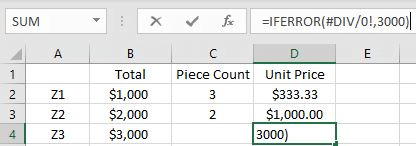This time expression B4/C4 returns an error #DIV/0!, IFERROR detects this error and comes to value_if_error, we set value_if_error to B4, so value 3000 in B4 is returned by this formula.

### RETURN A TEXT

We can replace error value with some other values. For example, we can enter text “ERROR” in the formula, if error is detected by Excel IFERROR function, “ERROR” is recorded in unit price column.

The formula is:

`=IFERROR(B2/C2,"ERROR")`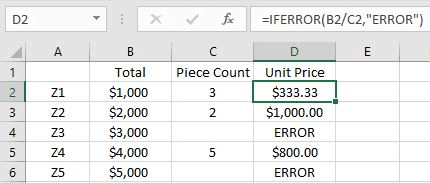Notes:

If text is entered in a formula, the entered text must be enclosed in double quotation marks. Otherwise, an error #NAME? will be thrown.

### Related Functions

• Excel IFERROR function
The Excel IFERROR function returns an alternate value you specify if a formula results in an error, or returns the result of the formula.The syntax of the IFERROR function is as below:= IFERROR (value, value_if_error)….

Related Posts

Find and Replace Multiple Values

This post will guide you how to find and replace multiple values at once with VBA macro or using formula  in Excel. How do I make multiple find and replace in Excel. Suppose that you have a few cells containing ...

Excel XLOOKUP Function

Excel XLOOKUP Function was added into Excel as a beta feature in August 2019 and is now accessible exclusively in Microsoft 365. (as of July 2021). However, if you fall into this category and often deal with big sets of ...

Extract matching values From Two Lists

Suppose that you are working with two lists containing few values, and you want to extract the matching values from those two lists into another separate list. You might prefer to manually extract the matching values from the two lists, ...

Excel Filter Function (10 Examples)

This post will guide you how to extracts matched values using FILTER function in Microsoft Excel 365. And also will introduce that how to use FILTER function with same examples in Excel 365. Excel Filter Function The FILTER function "filters" ...

Extract Multiple Match Values into Separate Columns

If you have a few values/items in the excel sheet and you are thinking that with the aid of the “VlOOKUP” function you can look for a specific value, extract it and then put the matching item into the separate ...

Sidebar